Courses

# Test: Simple Stress & Strain Level - 2

## 20 Questions MCQ Test Strength of Materials (SOM) | Test: Simple Stress & Strain Level - 2

Description
This mock test of Test: Simple Stress & Strain Level - 2 for Mechanical Engineering helps you for every Mechanical Engineering entrance exam. This contains 20 Multiple Choice Questions for Mechanical Engineering Test: Simple Stress & Strain Level - 2 (mcq) to study with solutions a complete question bank. The solved questions answers in this Test: Simple Stress & Strain Level - 2 quiz give you a good mix of easy questions and tough questions. Mechanical Engineering students definitely take this Test: Simple Stress & Strain Level - 2 exercise for a better result in the exam. You can find other Test: Simple Stress & Strain Level - 2 extra questions, long questions & short questions for Mechanical Engineering on EduRev as well by searching above.
QUESTION: 1

### The modulus of rigidity of an elastic material is found to be 38.5% of the value of its young’s modulus. The Poisson’s ratio μ of the material is nearly

Solution: The relationship between elastic constants of material,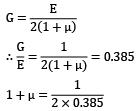μ = 0.298

QUESTION: 2

### For a material following Hooke’s law, the values of elastic and shear moduli are 3 x 105 and 1.2 x 105 MPa respectively. The value for bulk modulus is

Solution: E = 3 x 105 MPa G = 1.2 x 105 MPa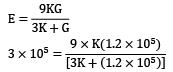3K + (1.2 x 105) = 3.6K

0.6K = 1.2 x 105

K = 2 x 105 MPa

QUESTION: 3

### The modulus of rigidity and the bulk modulus of a material are found as 70GPa and 150GPa respectively. Then1. elasticity modulus is 200GPa2. Poisson’s ratio is 0.223. elasticity modulus is 182GPa4. Poisson’s ratio is 0.3Which of the above statements are correct?

Solution: G = 70 GPa K = 150 GPa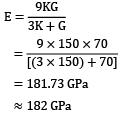= 181.73 GPa ≈ 182 GPa

E = 2G (1 + μ)

182 = (2 x 70)(1 + μ)

μ = 0.3

QUESTION: 4

If the ratio G/E (G = rigidity modulus, E = Young’s modulus of elasticity) is 0.4, then what is the value of the Poission’s ratio?

Solution: E = 2G (1 + μ)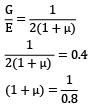1 + μ = 1.25

μ = 0.25

QUESTION: 5

The modulus of elasticity for a material is 200 GN/m2 and Poisson’s ratio 0.25 What is the modulus of rigidity?

Solution: Given, E = 200 GN/m2

μ = 0.25

E = 2G (1 + μ)

⇒ 200 = 2G(1.25)

G = 80 GN/m2

QUESTION: 6

If E, G and K denote Young’s modulus, modulus of rigidity and bulk modulus, respectively, for an elastic material, then which one of the following can be possible true?

Solution: For elastically material, poisons ratio lies between 1/4and 1/3.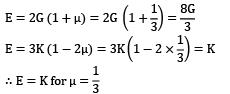QUESTION: 7

If a piece of material neither expands or contracts in volume when subjected to stresses, then the Poission’s ratio must be

Solution: Volumetric strain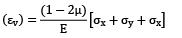Material neither expands nor contracts in volume, so

εv = 0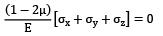1 − 2μ = 0

μ = 0.5

QUESTION: 8

A copper rod 400mm long is pulled in tension to a length of 401.2mm by applying a tensile load of 330MPa. If the deformation is entirely elastic, the Young’s modulus of copper is

Solution: σ = 330 MPa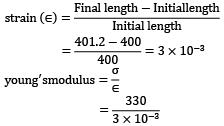= 110 x 103 MPa

= 110 GPa

QUESTION: 9

The deformation of a bar under its own weight as compared to that when subjected to a direct axial load equal to its own weight will be

Solution: Deformation under its own weight,

δ1 = WL/2AE

Deformation due to axial load w, δ2 = WL / AE

⇒ δ2 = 2δ1

or

δ1 = 1 / 2δ2

QUESTION: 10

A steel rod 10 m long is at a temperature of 20℃. The rod is heated to a temperature of 60℃. What is the stress induced in the rod if it is allowed to expand by 4mm, when E = 200GPa and α = 12 x 10−6/℃?

Solution: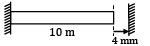ΔT = 60°C − 20°C = 40°C

E = 200 GPa

α = 12 x 10−6/°C

For expansion in rod = L ∙ α ∙ ΔT

= 10 x 103 x 12 x 10−6 x 40

= 4.8 mm

Net ΔLx = 0.8 mm compression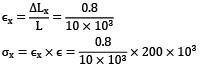= 16 MPa

QUESTION: 11

A circular steel rod of 20 cm2 cross-sectional area and 10m length is heated through 50℃ with ends clamped before heating. Given, E = 200 GPa and coefficient of thermal expansion, α = 10 x 10−6 /℃, the thrust thereby generated on the clamp is

Solution: The thermal strain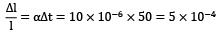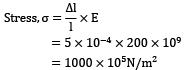Force developed, F = σ. A = 1000 x 105 x 20 x 10−4

= 20 x 108−4

= 20 x 104 N

= 200 kN

QUESTION: 12

A copper rod of 2 cm diameter is completely encased in a steel tube of inner diameter 2cm and outer diameter 4cm. Under an axial load, the stress in the steel tube is 100 N/mm2. If Es = 2Ec , then the stress in the copper rod is

Solution: For copper, d = 2 cm = 20 mm For steel,d1 = 2 cm, do = 4 cm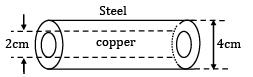σs = 100N/mm2

Es = 2Ec

Strain in steel = strain in copper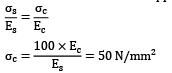QUESTION: 13

A bar produces a lateral strain of magnitude 60 x 10−5 when subjected to a tensile stress of magnitude 300 MPa along the axial direction. The elastic modulus of the material if the Poisson’s ratio is 0.3 is

Solution: Lateral strain = Axial strain x poisson′ s ratio ∈y=∈x x μ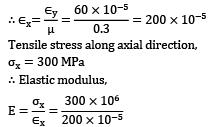= 150 GPa

QUESTION: 14

A cube with a side length of 1 cm is heated uniformly 1℃ above the room temperature and all the sides are free to expand. What will be the increase in volume of the cube? (Given coefficient of thermal expansion is α per ℃)

Solution: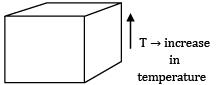Volumetric strain, εv = εx + εy + εz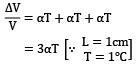⇒ ∆V = 13 x 3α = 3α cm3

QUESTION: 15

A metal rod is rigidly fixed at its both ends. The temperature of the rod has increased by 100℃. If the coefficient of linear expansion and elastic modulus of the metal rod are 10 x 10−6/℃ and 200GPa respectively, then what is the stress produced in the rod?

Solution: α = 10 x 10-6/0C

∆t = 100℃

E = 200 GPa = 200 x 103 MPa

If the temperature of the rod is increased, the rod will try to elongate. But due to rigid fixed support, it will not elongate and compressive stress acts on it.

σ = Eα∆t = 200 x 103 x 10 x 10−6 x 100

= 200 MPa (compressive)

QUESTION: 16

During tensile-testing of a specimen using a Universal Testing Machine, the parameters actually measured include

Solution:
QUESTION: 17

If the area of cross-section of a wire is circular and if the radius of this circle decreases to half its original value due to the stretch of the wire by a load, then the modulus of elasticity of the wire be

Solution: Young’s modulus is constant for a given material

QUESTION: 18

A tension member of square cross-section of side 10 mm and Young’s modulus E is to be replaced by another member of square cross-section of same length but young’s modulus E/2. The side of the new square cross-section, required to maintain the same elongation under the same load, is nearly

Solution: Member 1,

Area(A1) = 10 x 10mm2 = 100mm2

E1 = E

Member2,

E2 = E/2

A2 =?

Given , P1 = P2: and L1 = L2 and δ1 = δ2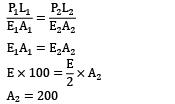If a is the length of the side of square

a2 = 200

a = 14.142 mm

QUESTION: 19

A rod of length l tapers uniformly from a diameter D at one end to a diameter D/2 at the other end and is subjected to an axial load P. A second rod of length l and of uniform diameter D is subjected to the same axial load P. Both the rods are of same material with Young’s Modulus of elasticity E. The ratio of extension of the first rod to that of the second rod is

Solution: Extension of the tapered rod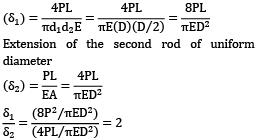Extension of the second rod of uniform diameterQUESTION: 20

Assertion(A): Poisson’s ratio of a material is a measure of its ductility

Reason(R): For every linear strain in the direction of force, Poisson’s ratio of the material gives the lateral strain in directions perpendicular to the direction of force

Solution: